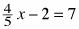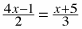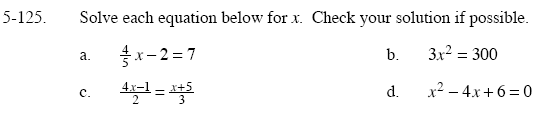### Home > GC > Chapter 5 > Lesson 5.3.5 > Problem5-125

5-125.
1. Solve each equation below for x. Check your solution if possible. Homework Help ✎

1.2. 3x2 = 300

3.4. x2 − 4x + 6 = 0Multiply by 5 to make the equation easier to solve.

You should have two solutions.

3(4x – 1) = 2(x + 5)

Solve for x using the Quadratic Formula.